# Trigonometry facts for kids

Kids Encyclopedia Facts

Trigonometry (from the Greek trigonon = three angles and metron = measure) is a part of elementary mathematics dealing with angles, triangles and trigonometric functions such as sine (abbreviated sin), cosine (abbreviated cos) and tangent (abbreviated tan). It has some connection to geometry, although there is disagreement on exactly what that connection is; for some, trigonometry is just a section of geometry.

## Overview and definitions

Trigonometry uses a large number of specific words to describe parts of a triangle. Some of the definitions in trigonometry are:

• Right-angled triangle - A right-angled triangle is a triangle that has an angle equal to 90 degrees. (A triangle cannot have more than one right angle) The standard trigonometric ratios can only be used on right-angled triangles.
• Hypotenuse - The hypotenuse of a triangle is the longest side, and the side that is opposite the right angle. For example, for the triangle on the right, the hypotenuse is side c.
• Opposite of an angle - The opposite side of an angle is the side that does not intersect with the vertex of the angle. For example, side a is the opposite of angle A in the triangle to the right.
• Adjacent of an angle - The adjacent side of an angle is the side that intersects the vertex of the angle but is not the hypotenuse. For example, side b is adjacent to angle A in the triangle to the right.

## Trigonometric ratios

There are three main trigonometric ratios for right triangles, and three reciprocals of those ratios, making up a total of 6 ratios. They are:

• Sine (sin) - The sine of an angle is equal to the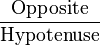$\textstyle \text{Opposite} \over \text{Hypotenuse}$.
• Cosine (cos) - The cosine of an angle is equal to the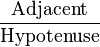$\textstyle \text{Adjacent} \over \text{Hypotenuse}$.
• Tangent (tan) - The tangent of an angle is equal to the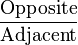$\textstyle \text{Opposite} \over \text{Adjacent}$.

The reciprocals of these ratios are:

Cosecant (csc) - The cosecant of an angle is equal to the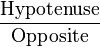$\textstyle \text{Hypotenuse} \over \text{Opposite}$ or$\csc \theta = {1 \over \sin \theta}$.

Secant (sec) - The secant of an angle is equal to the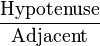${\text{Hypotenuse} \over \text{Adjacent}}$ or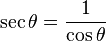$\sec \theta = {1 \over \cos \theta}$.

Cotangent (cot) - The cotangent of an angle is equal to the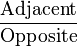${\text{Adjacent} \over \text{Opposite}}$ or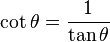$\cot \theta = {1 \over \tan \theta}$.

Students often use a mnemonic to remember this relationship. The sine, cosine, and tangent ratios in a right triangle can be remembered by representing them as strings of letters, such as SOH-CAH-TOA:

Sine = Opposite ÷ Hypotenuse

## Using trigonometry

With the sines and cosines, one can answer virtually all questions about triangles. This is called "solving" the triangle. One can work out the remaining angles and sides of any triangle, as soon as two sides and their included angle or two angles and a side or three sides are known. These laws are useful in all branches of geometry, since every polygon may be described as a combination of triangles.

Trigonometry is also vital in surveying, in vector analysis, and in the study of periodic functions. It developed from a need to compute angles and distances in fields such as astronomy, mapmaking, surveying, and artillery range finding.

There is also such a thing as spherical trigonometry, which deals with spherical geometry. This is used for calculations in astronomy, geodesy and navigation.

## Trigonometry Laws

### Law of Sines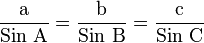${\text{a} \over \text{Sin A}}={\text{b} \over \text{Sin B}}={\text{c} \over \text{Sin C}}$

### Law of Cosines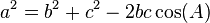$a^2=b^2+c^2-2bc \cos(A)$

### Law of Tangents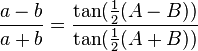$\frac{a-b}{a+b}=\frac{\tan(\frac{1}{2}(A-B))}{\tan(\frac{1}{2}(A+B))}$

## Images for kidsTrigonometry Facts for Kids. Kiddle Encyclopedia.